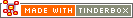^min( group , attribute[, precision] )^Code Type: Calculation Code Scope of Action: group

^min( group , attribute[, precision] )^

Returns the attribute value for the note within the group whose attribute value is the smallest.

An optional third argument lets you set the number of decimal points that are exported by the numeric group mark-up elements(^sum^, ^min^, ^max^, ^mean^, ^count^). Note that the precision is always the third argument.

For internal use, see the min() operator.

 Up: Calculation Previous: ^mean( group , attribute[, precision] )^ Next: ^sum( group , attribute[, precision] )^

[Last updated: 14 Dec 2009, using v5.0]